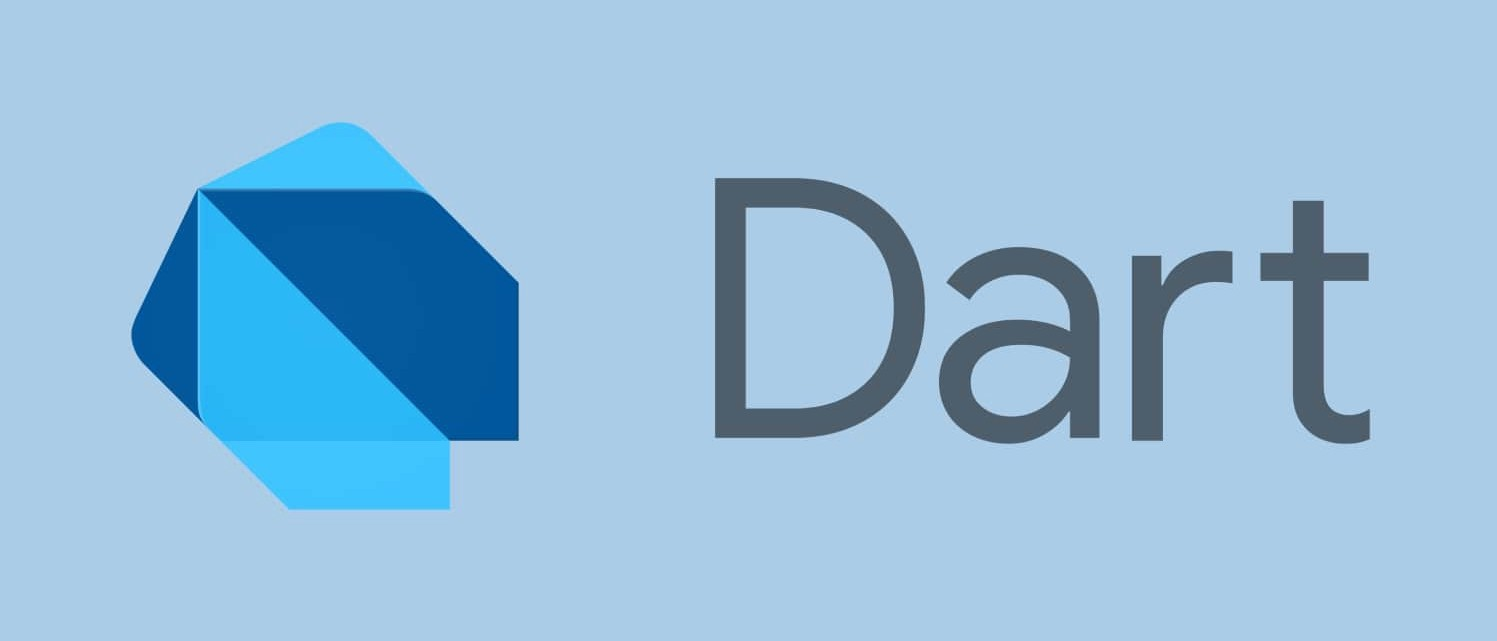### Integer in Dart #

In the previous video, We talked about the numbers in Dart. In this video I’ll talk about integer in dart.

How to define/declare a variable integer?main.dart
``````int variable_name;
``````

How to define/declare a variable integer with value?main.dart
``````int variable_name = 6;
``````

Once you declare a variable with it’s type name after that computer will remember that this label is of type integer or double or whatever type you have wrote in the program.

In dart `integer` is refereed as `int`

#### Example 1 #

In this example we will define/declare a variable `a` of int(integer number) type, after that we will assign some value to `a`.main.dart
``````int main(){
int a;
a = 2;
print(a);
}
``````

#### Example 2 #

Here I defined a variable `a` with the initial value of 2.main.dart
``````int main(){
int a = 2;
print(a);
}
``````

### Operation on Numeric #

There are various operation which can be performed on Number.

What operation can be performed on Numbers. You can almost perform all the mathematical operation on the number.

## Basic Mathematics Operation #

• Difference (Subtract)
• Product (Multiply)
• Division

#### Sum of Integer #main.dart
``````int main(){
int a = 12;
int b = 4;
int c = a + b;
print(c);   // Result will be 16
}
``````

#### Difference of Integer #main.dart
``````int main(){
int a = 12;
int b = 4;
int c = a - b;
print(c);   // Result will be 8
}
``````

#### Product of Integer #main.dart
``````int main(){
int a = 12;
int b = 4;
int c = a * b;
print(c);   // Result will be 48
}
``````

#### Division of Integer #main.dart
``````int main(){
int a = 12;
int b = 4;
double c = a / b;
print(c);   // Result will be 3
}
``````